# MCAT Physical : Voltage, Energy, and Power

## Example Questions

← Previous 1

### Example Question #1 : Voltage, Energy, And Power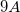current flows through a wire with a resistance of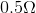. Find the power generated by this current.

Possible Answers: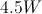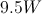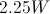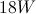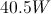Correct answer:Explanation:

Electric power is expressed in a number of ways. We will need to use the relationship that involves current and resistance: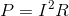We are given the current and resistance, allowing us to calculate the power.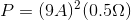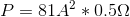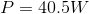### Example Question #2 : Voltage, Energy, And Power

A capacitor attached to a 50 V battery aquires a charge of 10 C over a period of 5 seconds. How much energy is required to charge the capacitor?

Possible Answers:

50 J

250 J

1250 J

500 J

Correct answer:

1250 J

Explanation:

The power consumed by a capacitor is given by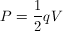After finding power, we can calculate the energy used as we are told that the capacitor took 5 seconds to charge. The units of power are watts which can be further broken down into joules per second. By multiplying the time taken to charge the capacitor by the power, we can find the total energy required to charge the capacitor.

### Example Question #3 : Voltage, Energy, And Power

A low resistance light bulb and a high resistance light bulb are connected in parallel with each other. This parallel combination is in series with a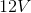battery. Which bulb is brighter?

Possible Answers:

Only the high resistance bulb will be lit

Both bulbs are equally bright

Only the low resistance bulb will be lit

The low resistance bulb

The high resistance bulb

Correct answer:

The low resistance bulb

Explanation:

The brightness of each bulb depends on its power, or how much energy it dissipates per unit time. This can be calculated using the equation: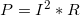If the bulbs are in parallel with each other, the bulb with lower resistance will receive more current, since current will tend toward the path of least resistance. Current is squared in the power equation, so it is of greater importance in determining the power. The bulb with greater current and lower resistance will thus have more power and will shine more brightly.

### Example Question #4 : Voltage, Energy, And Power

During the cold winter months, some gloves have the ability to provide extra warmth due to an internal heating source. A simplified circuit, similar to those in electric gloves, is comprised of a 9V battery with no internal resistance and three resistors as shown in the image below.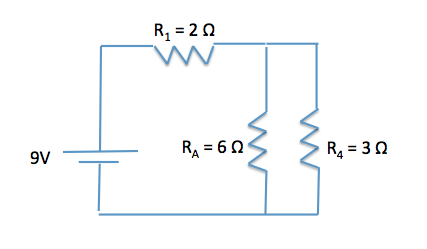How much power does the circuit provide?

Possible Answers: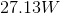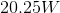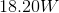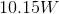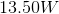Correct answer:Explanation:

This question asks us about the power of the circuit, meaning the amount of energy per unit time. The power equation is P = IV. V = IR can be substituted in to allow P to be calculated from a number of parameters.

P = IV = I2R = V2/R

To solve for P, we first need the current supplied by the battery.

We can use the formula V = IR because we have the voltage drop across the circuit (9V) and can calculate the equivalent resistance.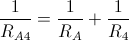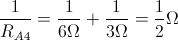By taking the inverse of the equation, we can see that RA4 is equal to 2Ω.

Req = RA4 + R1 = 2Ω + 2Ω = 4Ω

Now, using V=IR, we can solve for the current.

V = IR

I = V/R = 9V/4Ω = 2.25A

Now, we can use the current we calculated and the voltage that is dissipated across the circuit to calculate the power.

P = IV = (2.25 A)(9V) = 20.25W

### Example Question #5 : Voltage, Energy, And Power

What is the resistance in a wire carrying a voltage of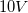and with a power of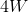?

Possible Answers: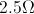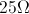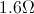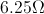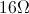Correct answer:Explanation:

The formula we can use here is the power formula that involved both resistance and voltage: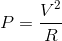We are given the voltage and power, allowing us to solve for the resistance.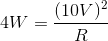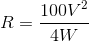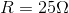### Example Question #6 : Voltage, Energy, And Power

Household voltage in the United States is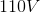, supplied by an alternating current (AC). What is the maximum voltage at the outlet?

Possible Answers: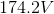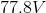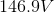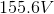Correct answer:Explanation:

Alternative current (AC) voltage is governed by the RMS (root-mean square) voltage law. This means that the RMS voltage of the circuit will be, but the actual voltage will fluctuate between two values. These values are determined by the equation: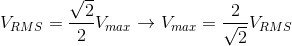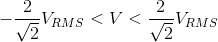Using the values from our question, we can find the maximum voltage value.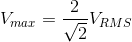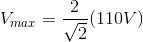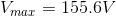### Example Question #7 : Voltage, Energy, And Power

During the cold winter months, some gloves have the ability to provide extra warmth due to an internal heating source. A simplified circuit, similar to those in electric gloves, is comprised of a 9V battery with no internal resistance and three resistors as shown in the image below.Instead of assuming that the 9V battery has no internal resistance, what would the terminal potential of the battery be if it has an internal resistance of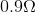?

Possible Answers: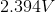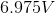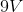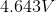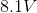Correct answer:Explanation:

Remember that internal resistance lowers the EMF of the battery. We need to calculate the voltage drop that would occur inside the battery, and then subtract that from the “stated” voltage to determine the terminal potential of the battery.

We first need the current supplied by the battery. We can use the formula V = IR because we have the voltage drop across the circuit (9V) and can calculate the equivalent resistance.By taking the inverse of the equation, we can see that RA4 is equal to 2Ω.

Req = RA4 + R1 = 2Ω + 2Ω = 4Ω

Now, using V=IR, we can find the current in the circuit.

V = IR

I = V/R = 9V/4Ω = 2.25A

Plugging in this value with the internal resistance of the battery gives us the voltage decrease.

V = IR = (2.25A)(0.9Ω) = 2.025V

Terminal Potential = 9V – 2.025V = 6.975V

As we can see, battery companies are interested in keeping the internal resistance of batteries at a minimum, because it lowers the overall terminal potential of the battery.

### Example Question #8 : Voltage, Energy, And Power

A circuit with two resistors (Resistor A and Resistor B) of equal resistance set up in parallel is attached to a battery with negligible internal resistance. Which of the following will occur if resistor B is removed?
(The wire of the circuit that resistor B is on will remain, however).

Possible Answers:

The voltage drop through resistor A will remain the same.

The voltage drop through resistor A will decrease.

The voltage drop through resistor A will increase.

The current through resistor A will increase.

Correct answer:

The voltage drop through resistor A will remain the same.

Explanation:

For this question we need to know that the voltage drop is equal through all paths of a parallel circuit; therefore we can realize that the voltage drop through both resistors are independent of each other. The current through resistor A will decrease with the removal of resistor B. Electrons want to travel through the path of least resistance.

### Example Question #9 : Voltage, Energy, And Power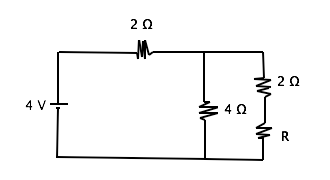Given that the resistance of resisitor R = 2Ω, what is the voltage drop across the 4 Ω resistor in the circuit shown above?

Possible Answers:

0 .666 V

3.33 V

2 V

4 V

1 V

Correct answer:

2 V

Explanation:

Given that resistor R = 2 Ω, we can see that the parallel aspect of the circuit has a resistance of 2 Ω as a whole, which means the total effective resistance of the circuit is 4 Ω. The potential difference drops 2V over the first resistor, then 2 V through the parallel aspect of the circuit. Potential difference, or voltage, drops through an equal amount in all branches of resistors in parallel. Therefore the remaining 2 V of potential difference is dropped by electrons moving through both branches of the parallel resistor, and the voltage drop through the 4 Ω resistor is 2V.### Example Question #10 : Voltage, Energy, And PowerWhat is the change in electrical potential energy for a charge of -2 µC moving through the circuit shown above?

Possible Answers:

–8 µJ

–2 µJ

16 µJ

2 µJ

8 µJ

Correct answer:

–8 µJ

Explanation:

Given that

V(q) = U

When V is voltage, U is electrical potential energy and q is charge, we can solve by plugging in 4 for V and -2 for q. Also, we must understand that the electric potential energy of a particle decreases as it moves from an area of higher energy to one of lower energy. For an electron, it has higher energy in the negative terminal of the battery than it does in when getting to the positive terminal.

← Previous 1

### All MCAT Physical Resources